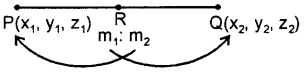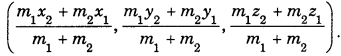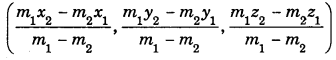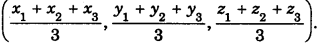By going through these CBSE Class 11 Maths Notes Chapter 12 Introduction to three Dimensional Geometry Class 11 Notes, students can recall all the concepts quickly.

## Introduction to three Dimensional Geometry Notes Class 11 Maths Chapter 12

Co-ordinates of a Point: The co-ordinates of a point are the distances from the origin of the feet of the perpendiculars from the point on the respective co-ordinate axes.

Distance Formula : The distance between the points (x1 y1, z1) and (x2, y2, z2) is given by $$\sqrt{\left(x_{2}-x_{1}\right)^{2}+\left(y_{2}-y_{1}\right)^{2}+\left(z_{2}-z_{1}\right)^{2}}$$. The distance of the point (x, y, z) from the origin is given by $$\sqrt{x^{2}+y^{2}+z^{2}}$$

Section Formulae: .
(i) Section formula for internal division :
If P(x1 y1, z1) and Q(x2, y2, z2) are two points. Let R be a point on the line segment P and Q such that it divides the join of P and Q internally in the ratio m1 : m2.Then, the co-ordinates of R are(ii) Section formula for external division :
If P(x1 y1, z1) and Q(x2, y2, z2) are two points and let R be a
point on PQ produced dividing it
externally in the ratio m1 : m2(m1 ≠ m2). Then, the co-ordinates of RareMid-Point : The mid-point of the line segment joining (x1 y1, z1) and (x2 y2, z2) is $$\left(\frac{x_{1}+x_{2}}{2}, \frac{y_{1}+y_{2}}{2}, \frac{z_{1}+z_{2}}{2}\right)$$

Centroid : Centroid of the triangle whose vertices are (x1 y1, z1), (x2 y2, z2) and (x3 y3, z3) is